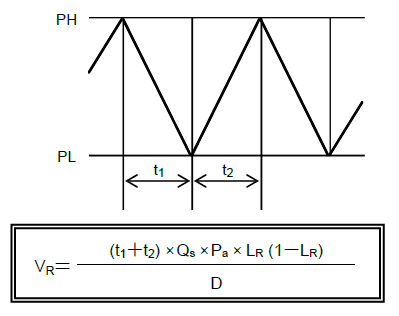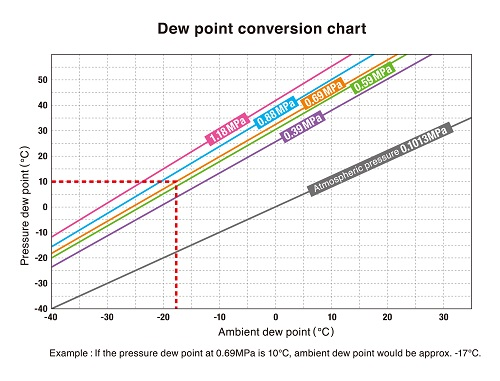## Required capacity of receiver tank

This is a calculation example for determining rough estimate of required receiver tank capacity.t1+t2=

Example: In case of SGIV, t1+t2 ≧ 0.5 min ≧(30 seconds or more)

Qs=

Discharge air flow of compressor

Pa=

Suction absolute pressure (atmospheric pressure = 0.101 Mpa)

D=

Difference between upper pressure limit (PH) and lower pressure limit (PL)

x=

Load ratio (air consumption rate against discharge air flow of compressor on above Qs)

* The tank capacity becomes maximum when the load ratio is 50%.

Vr=

Disclaimer: The calculation results are for reference only and we are not responsible for any results produced using the calculations.

## Selection of compressors for additional installations

This is a calculation example for determining a rough estimate of how much air flow rate must be increased by installing additional compressors, to maintain higher pressure when a large amount of air consumption is reducing pressure.

V2 ≧ V1 × (

P2＋0.1013

P1＋0.1013

-1)

P1

Current line pressure

P2

Desired sustained level of pressure

V1

Total air flow rate of existing compressors

V2=

Required air flow rate for additionally installed compressors

Disclaimer: The calculation results are for reference only and we are not responsible for any results produced using the calculations.

## Amount of drained water

The maximum amount of water vapor in the air (amount of saturated water vapor) increases as temperature elevates and decreases as pressure elevates. Compressing air, therefore, causes draining of water.

a

Discharge air flow of compressor

b

Compressor operating time

### 1) Moisture content in the air suctioned by compressor

Suctioned air temperature

c

Amount of saturated water vapor

d

Relative humidity

e=c*d

Moisture content in the suctioned air (per m3/min)

f=a*b*e*60/1000

Moisture content in the air suctioned by compressor

### 2) Moisture content in the output of dryer

Atmospheric dew point (refer to below chart for conversion from pressure dew point)

g

Amount of saturated water vapor

h=a*b*g*60/1000

Total moisture content in the output of dryer### 3) Amount of drained water at dryer output

f-h

Disclaimer: The calculation results are for reference only and we are not responsible for any results produced using the calculations.